# Data Structures and Files

Teacher
22 de Feb de 2018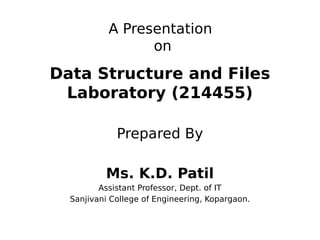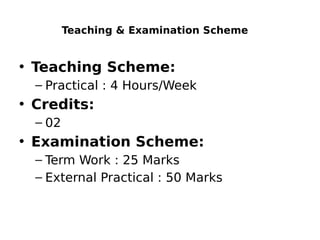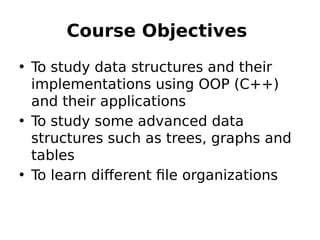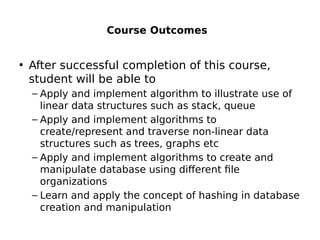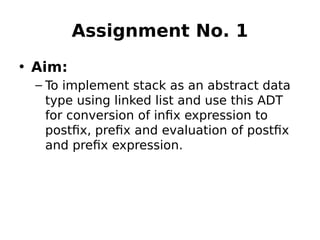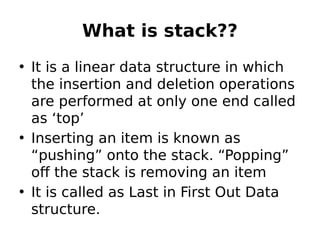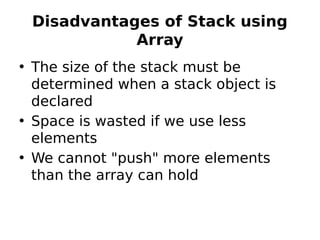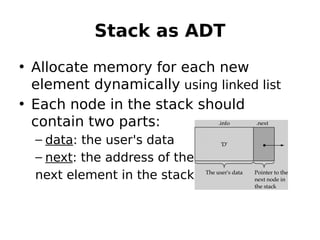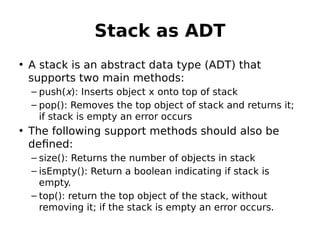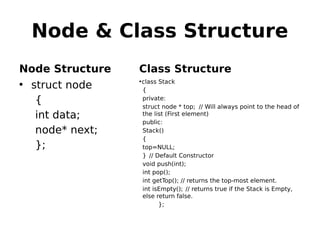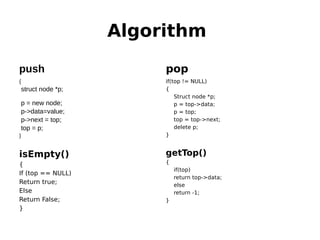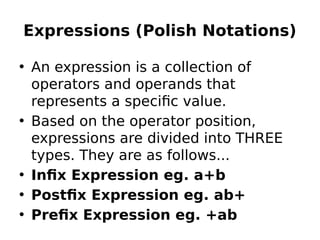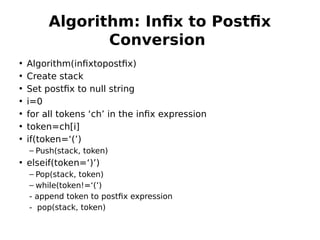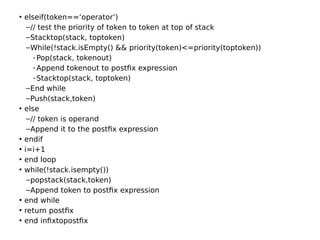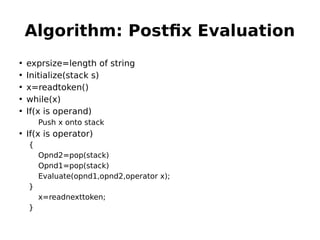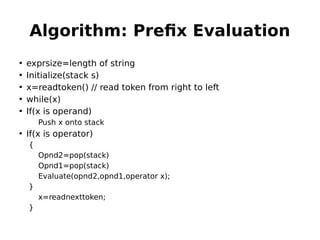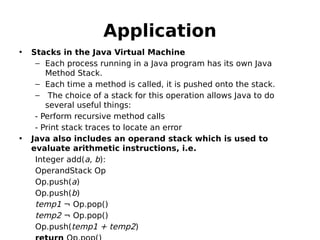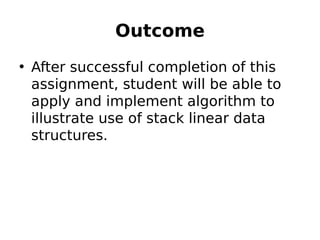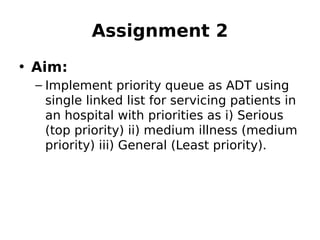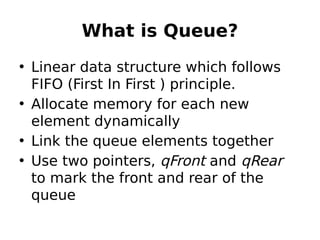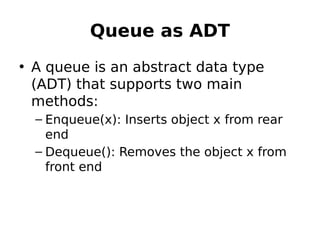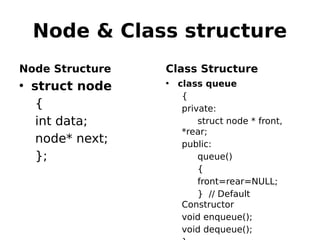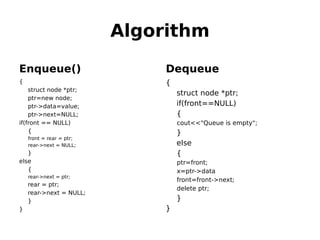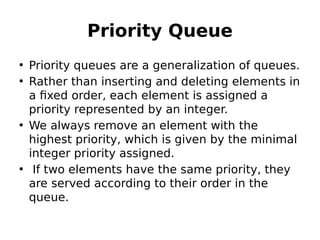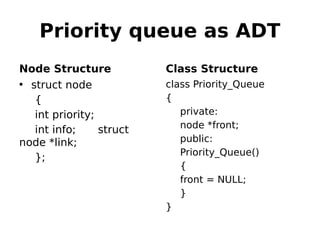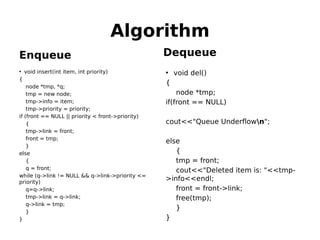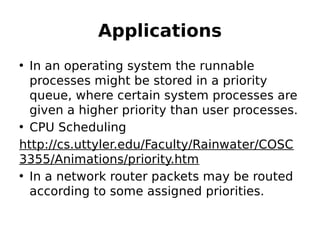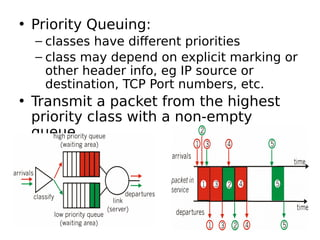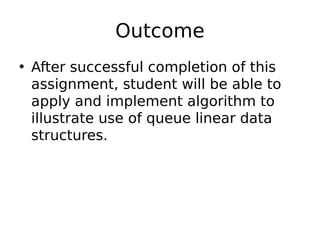1 de 29

### Data Structures and Files

• 1. A Presentation on Data Structure and Files Laboratory (214455) Prepared By Ms. K.D. Patil Assistant Professor, Dept. of IT Sanjivani College of Engineering, Kopargaon.
• 2. Teaching & Examination Scheme • Teaching Scheme: – Practical : 4 Hours/Week • Credits: – 02 • Examination Scheme: – Term Work : 25 Marks – External Practical : 50 Marks
• 3. Course Objectives • To study data structures and their implementations using OOP (C++) and their applications • To study some advanced data structures such as trees, graphs and tables • To learn different file organizations
• 4. Course Outcomes • After successful completion of this course, student will be able to – Apply and implement algorithm to illustrate use of linear data structures such as stack, queue – Apply and implement algorithms to create/represent and traverse non-linear data structures such as trees, graphs etc – Apply and implement algorithms to create and manipulate database using different file organizations – Learn and apply the concept of hashing in database creation and manipulation
• 5. Assignment No. 1 • Aim: – To implement stack as an abstract data type using linked list and use this ADT for conversion of infix expression to postfix, prefix and evaluation of postfix and prefix expression.
• 6. What is stack?? • It is a linear data structure in which the insertion and deletion operations are performed at only one end called as ‘top’ • Inserting an item is known as “pushing” onto the stack. “Popping” off the stack is removing an item • It is called as Last in First Out Data structure.
• 7. Disadvantages of Stack using Array • The size of the stack must be determined when a stack object is declared • Space is wasted if we use less elements • We cannot "push" more elements than the array can hold
• 8. Stack as ADT • Allocate memory for each new element dynamically using linked list • Each node in the stack should contain two parts: – data: the user's data – next: the address of the next element in the stack
• 9. Stack as ADT • A stack is an abstract data type (ADT) that supports two main methods: – push(x): Inserts object x onto top of stack – pop(): Removes the top object of stack and returns it; if stack is empty an error occurs • The following support methods should also be defined: – size(): Returns the number of objects in stack – isEmpty(): Return a boolean indicating if stack is empty. – top(): return the top object of the stack, without removing it; if the stack is empty an error occurs.
• 10. Node & Class Structure Node Structure • struct node { int data; node* next; }; Class Structure •class Stack { private: struct node * top; // Will always point to the head of the list (First element) public: Stack() { top=NULL; } // Default Constructor void push(int); int pop(); int getTop(); // returns the top-most element. int isEmpty(); // returns true if the Stack is Empty, else return false. };
• 11. Algorithm push { struct node *p; p = new node; p->data=value; p->next = top; top = p; } isEmpty() { If (top == NULL) Return true; Else Return False; } pop if(top != NULL) { Struct node *p; p = top->data; p = top; top = top->next; delete p; } getTop() { if(top) return top->data; else return -1; }
• 12. Expressions (Polish Notations) • An expression is a collection of operators and operands that represents a specific value. • Based on the operator position, expressions are divided into THREE types. They are as follows... • Infix Expression eg. a+b • Postfix Expression eg. ab+ • Prefix Expression eg. +ab
• 13. Algorithm: Infix to Postfix Conversion • Algorithm(infixtopostfix) • Create stack • Set postfix to null string • i=0 • for all tokens ‘ch’ in the infix expression • token=ch[i] • if(token=‘(’) – Push(stack, token) • elseif(token=‘)’) – Pop(stack, token) – while(token!=‘(’) - append token to postfix expression - pop(stack, token)
• 14. • elseif(token==‘operator’) –// test the priority of token to token at top of stack –Stacktop(stack, toptoken) –While(!stack.isEmpty() && priority(token)<=priority(toptoken)) -Pop(stack, tokenout) -Append tokenout to postfix expression -Stacktop(stack, toptoken) –End while –Push(stack,token) • else –// token is operand –Append it to the postfix expression • endif • i=i+1 • end loop • while(!stack.isempty()) –popstack(stack,token) –Append token to postfix expression • end while • return postfix • end infixtopostfix
• 15. Algorithm: Postfix Evaluation • exprsize=length of string • Initialize(stack s) • x=readtoken() • while(x) • If(x is operand) Push x onto stack • If(x is operator) { Opnd2=pop(stack) Opnd1=pop(stack) Evaluate(opnd1,opnd2,operator x); } x=readnexttoken; }
• 16. Algorithm: Prefix Evaluation • exprsize=length of string • Initialize(stack s) • x=readtoken() // read token from right to left • while(x) • If(x is operand) Push x onto stack • If(x is operator) { Opnd2=pop(stack) Opnd1=pop(stack) Evaluate(opnd2,opnd1,operator x); } x=readnexttoken; }
• 17. Application • Stacks in the Java Virtual Machine – Each process running in a Java program has its own Java Method Stack. – Each time a method is called, it is pushed onto the stack. – The choice of a stack for this operation allows Java to do several useful things: - Perform recursive method calls - Print stack traces to locate an error • Java also includes an operand stack which is used to evaluate arithmetic instructions, i.e. Integer add(a, b): OperandStack Op Op.push(a) Op.push(b) temp1 ¬ Op.pop() temp2 ¬ Op.pop() Op.push(temp1 + temp2)
• 18. Outcome • After successful completion of this assignment, student will be able to apply and implement algorithm to illustrate use of stack linear data structures.
• 19. Assignment 2 • Aim: – Implement priority queue as ADT using single linked list for servicing patients in an hospital with priorities as i) Serious (top priority) ii) medium illness (medium priority) iii) General (Least priority).
• 20. What is Queue? • Linear data structure which follows FIFO (First In First ) principle. • Allocate memory for each new element dynamically • Link the queue elements together • Use two pointers, qFront and qRear to mark the front and rear of the queue
• 21. Queue as ADT • A queue is an abstract data type (ADT) that supports two main methods: – Enqueue(x): Inserts object x from rear end – Dequeue(): Removes the object x from front end
• 22. Node & Class structure Node Structure • struct node { int data; node* next; }; Class Structure • class queue { private: struct node * front, *rear; public: queue() { front=rear=NULL; } // Default Constructor void enqueue(); void dequeue();
• 23. Algorithm Enqueue() { struct node *ptr; ptr=new node; ptr->data=value; ptr->next=NULL; if(front == NULL) { front = rear = ptr; rear->next = NULL; } else { rear->next = ptr; rear = ptr; rear->next = NULL; } } Dequeue { struct node *ptr; if(front==NULL) { cout<<"Queue is empty"; } else { ptr=front; x=ptr->data front=front->next; delete ptr; } }
• 24. Priority Queue • Priority queues are a generalization of queues. • Rather than inserting and deleting elements in a fixed order, each element is assigned a priority represented by an integer. • We always remove an element with the highest priority, which is given by the minimal integer priority assigned. •  If two elements have the same priority, they are served according to their order in the queue.
• 25. Priority queue as ADT Node Structure • struct node { int priority; int info; struct node *link; }; Class Structure class Priority_Queue { private: node *front; public: Priority_Queue() { front = NULL; } }
• 26. Algorithm Enqueue • void insert(int item, int priority) { node *tmp, *q; tmp = new node; tmp->info = item; tmp->priority = priority; if (front == NULL || priority < front->priority) { tmp->link = front; front = tmp; } else { q = front; while (q->link != NULL && q->link->priority <= priority) q=q->link; tmp->link = q->link; q->link = tmp; } } Dequeue • void del() { node *tmp; if(front == NULL) cout<<"Queue Underflown"; else { tmp = front; cout<<"Deleted item is: "<<tmp- >info<<endl; front = front->link; free(tmp); } }
• 27. Applications • In an operating system the runnable processes might be stored in a priority queue, where certain system processes are given a higher priority than user processes. • CPU Scheduling http://cs.uttyler.edu/Faculty/Rainwater/COSC 3355/Animations/priority.htm • In a network router packets may be routed according to some assigned priorities.
• 28. • Priority Queuing: – classes have different priorities – class may depend on explicit marking or other header info, eg IP source or destination, TCP Port numbers, etc. • Transmit a packet from the highest priority class with a non-empty queue
• 29. Outcome • After successful completion of this assignment, student will be able to apply and implement algorithm to illustrate use of queue linear data structures.